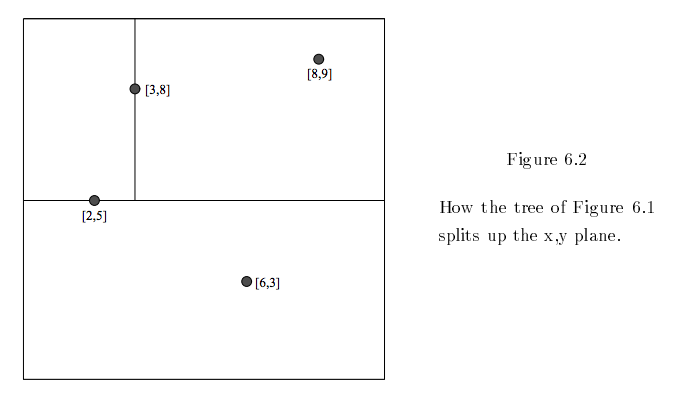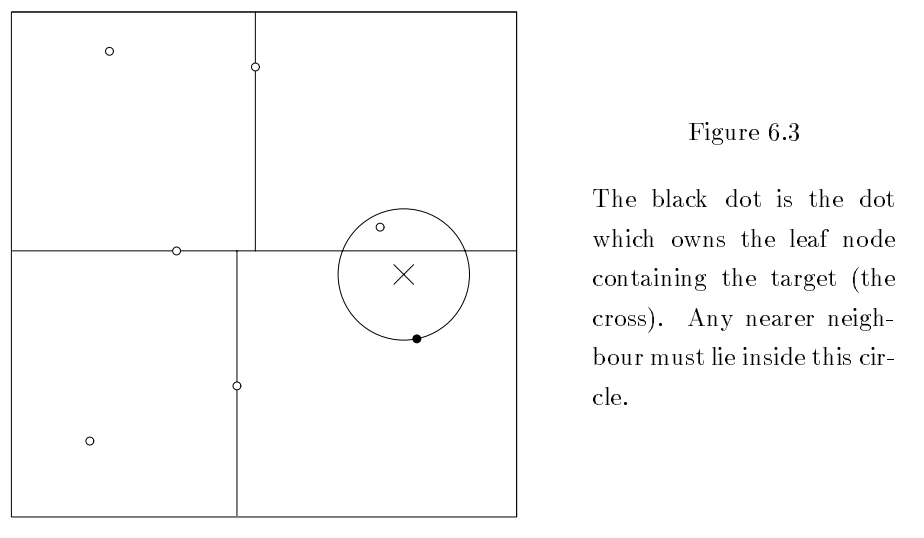# A Beginner's Guide to Unsupervised Learning

Unsupervised learning, in the field of machine learning, refers to learning without a ground truth such as labels to correct the error your model makes when guessing. An algorithm can learn in an unsupervised fashion, for example, by making a guess about the distribution of the data based on a sample, and then checking its guess against the actual distribution.

Unlike supervised learning, with unsupervised learning, we are working without a labeled dataset. What we generally learn, in the absence of a label, is how to reconstruct the input data using a representation, or embedding. Given the scarcity of labeled data in the world, the corrolary that supervised learning cannot be applied to most data, and the fact that models learn best when trained on more data – the potential for unsupervised learning on datasets without labels is enormous. The future of AI in large part depends on getting better at unsupervised learning.

Contents

The features learned by deep neural networks can be used for the purposes of classification, clustering and regression.

Neural nets are simply universal approximators using non-linearities. They produce “good” features by learning to reconstruct data through pretraining or through backpropagation. In the latter case, neural nets plug into arbitrary loss functions to map inputs to outputs.

The features learned by neural networks can be fed into any variety of other algorithms, including traditional machine-learning algorithms that group input, softmax/logistic regression that classifies it, or simple regression that predicts a value.

So you can think of neural networks as feature-producers that plug modularly into other functions. For example, you could make a convolutional neural network learn image features on ImageNet with supervised training, and then you could take the activations/features learned by that neural network and feed it into a second algorithm that would learn to group images.

Here is a list of use cases for features generated by neural networks:

## Visualization

t-distributed stochastic neighbor embedding (T-SNE) is an algorithm used to reduce high-dimensional data into two or three dimensions, which can then be represented in a scatterplot. T-SNE is used for finding latent trends in data. For more information and downloads, see this page on T-SNE.

## K-Means Clustering

K-Means is an algorithm used for automatically labeling activations based on their raw distances from other input in a vector space. There is no target or loss function; k-means picks so-called centroids. K-means creates centroids through a repeated averaging of all the data points. K-means classifies new data by its proximity to a given centroid. Each centroid is associated with a label. This is an example of unsupervised learning (learning lacking a loss function) that applies labels.

## Transfer Learning

Transfer learning takes the activations of one neural network and puts them to use as features for another algorithm or classifier. For example, you can take the model of a ConvNet trained on ImageNet, and pass fresh images through it into another algorithm, such as K-Nearest Neighbor. The strict definition of transfer learning is just that: taking the model trained on one set of data, and plugging it into another problem.

## K-Nearest Neighbors

This algorithm serves the purposes of classification and regression, and relies on a kd-tree. A kd-tree is a data structure for storing a finite set of points from a k-dimensional space. It partitions a space of arbitrary dimensions into a tree, which may also be called a vantage point tree. kd-trees subdivide a space with a tree structure, and you navigate the tree to find the closest points. The label associated with the closest points is applied to input.

Let your input and training examples be vectors. Training vectors might be arranged in a binary tree like so:If you were to visualize those nodes in two dimensions, partitioning space at each branch, then the kd-tree would look like this:Now, let’s saw you place a new input, X, in the tree’s partitioned space. This allows you to identify both the parent and child of that space within the tree. The X then constitutes the center of a circle whose radius is the distance to the child node of that space. By definition, only other nodes within the circle’s circumference can be nearer.And finally, if you want to make art with kd-trees, you could do a lot worse than this:(Hat tip to Andrew Moore of CMU for his excellent diagrams.)

### Other methods

In natural language processing, using words to predict their contexts, with algorithms like word2vec, is a form of unsupervised learning.